# VBA迴圈控制與If Then條件判斷，計算健保負擔金額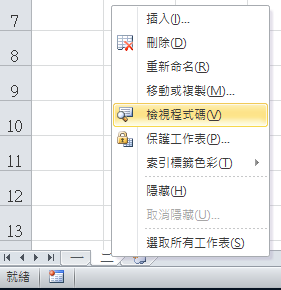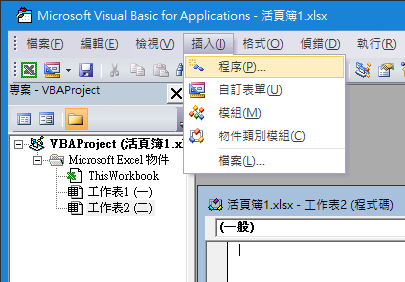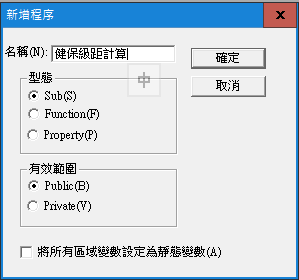Public Sub 健保級距計算（）

For i = 2 To 6 If Cells(i, “D”) < 20100 Then Cells(i, “G”) = 284

If Cells(i, “D”) >= 20100 And Cells(i, “D”) < 21000 Then Cells(i, “G”) = 296

If Cells(i, “D”) >= 21000 And Cells(i, “D”) < 21900 Then Cells(i, “G”) = 309

If Cells(i, “D”) >= 21900 And Cells(i, “D”) < 22800 Then Cells(i, “G”) = 323

If Cells(i, “D”) >= 22800 And Cells(i, “D”) < 24000 Then Cells(i, “G”) = 336

Next

End Sub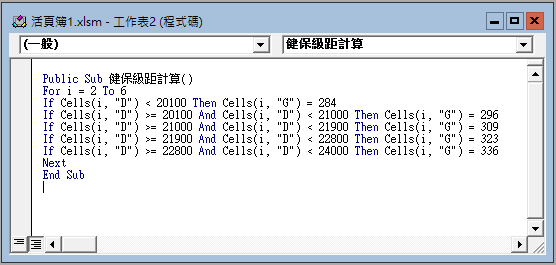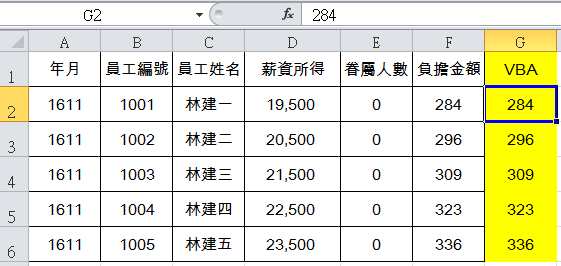Visual Basic for Applications，聽起來艱澀，似乎專屬於資工系資管系的工程師領域，然而以這一節的VBA範例來看，即便不多作說明，應該也能看得懂這幾段程式在「講」什麼，這是BASIC語言自1960s年代延續下來的特色，接近自然語言，因此容易理解、也便於學習。套句周爺老電影台詞：「只要有心，人人都可以是食神，」在Excel世界裡，只要有心，人人都可以讓VBA飛！